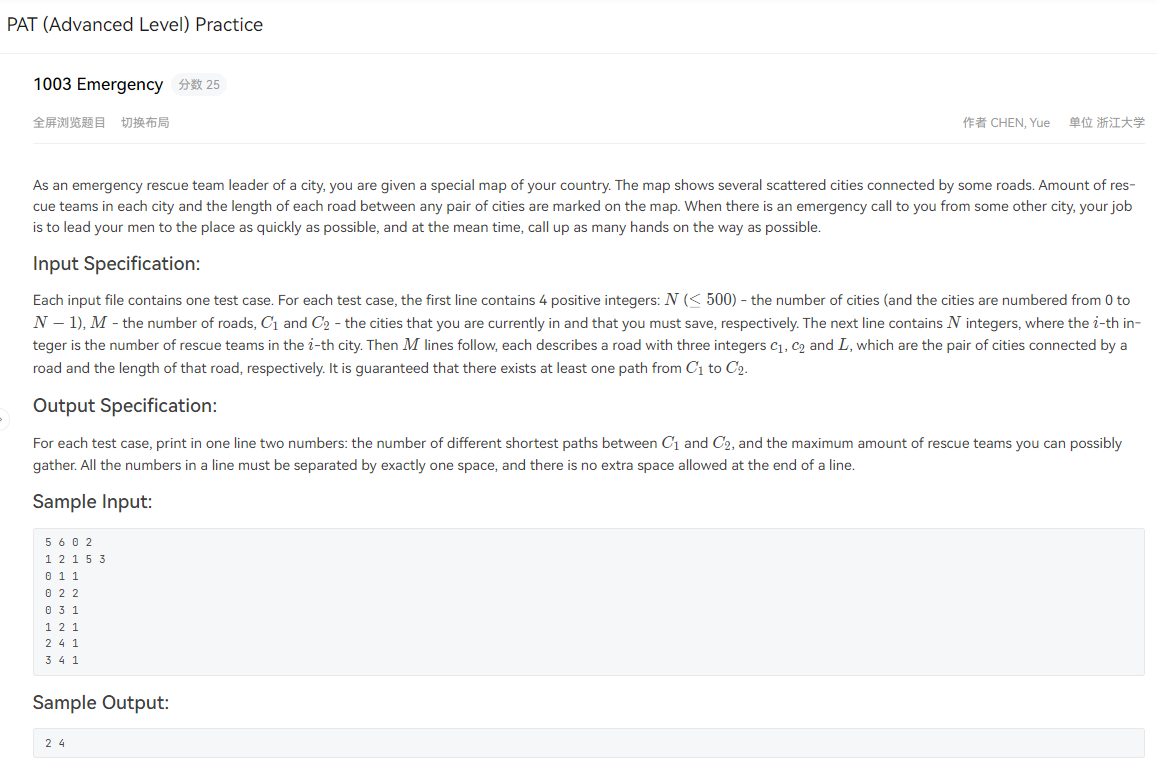# 题目介绍# 解题思路

​ 本题的大意是在给定起点和终点的情况下，要求你从一个无向图中找到是一条最短且尽可能多的救援队的路径。这是一个典型的求单源最短路径的题目，即使用`Dijkstra算法`，但这个题还要求了尽可能多的救援队的数量，也就是说如果最短路径不止一条你得在这些最短路径中找到救援队最多的那一条。

​ 很明显这是一个拥有第二标尺的dijkstra算法，第一标尺就是边权，第二标尺就是点权，即每个城市所用的救援队的数量。

​ 首先我们需要一个矩阵来存放路径，即图的边，然后用数组d，数组w，数组weight和数组num分别来存放源点到各个顶点的距离，源点到各个顶点的最大点权（是在满足最短路径的条件下），初始每个城市的救援队数量（初始点权）和从源点出发到各个顶点的最短路径数量。然后就是vis标记数组（老朋友了）。

​ dijkstra算法核心代码的含义写在了代码注释中，大家可以放心食用。

# 实现代码

• 邻接矩阵版本
``````#include<iostream>
#include<cstring>
#include<cstdlib>
#include<cstdio>
#include<vector>
#include<queue>
#include<sstream>
#include<algorithm>
using namespace std;

constexpr auto INF=0x3fffffff;
constexpr auto MAX=501;

int d[MAX],w[MAX],weight[MAX],num[MAX];
int G[MAX][MAX];
void Dijkstra(int origin);
bool vis[MAX]={false};
int edge,vertex,origin,destin;

int main()
{
#ifdef ONLINE_JUDGE
#else
freopen("1.txt", "r", stdin);
#endif

fill(G,G+MAX*MAX,INF);

cin>>vertex>>edge>>origin>>destin;

for(int i=0;i<vertex;++i)
{
cin>>weight[i];
}

int a,b,c;
for(int i=0;i<edge;++i)
{
cin>>a>>b>>c;
G[a][b]=G[b][a]=c;
}

//到这里一个无向图就好了，想一下有向图可不可以，评论可以留言一波哈！！！（不可以，必须是无向图，这也是4号测试点）
//dijkstra算法入口
Dijkstra(origin);

cout<<num[destin]<<" "<<w[destin];

system("pause");
return 0;
}

void Dijkstra(int origin)
{
//初始化，这里初始化要注意memset慎用
fill(d,d+MAX,INF);
fill(num,num+MAX,0);
fill(w,w+MAX,0);

d[origin]=0;

//这个地方说明一下为什么给1，因为不管怎么样，起点-终点，有且至少有一条最短路径，就算一个图只有一个点，即顶点和终点重合，也有一条最短路径，所以不肯能是0条
num[origin]=1;
w[origin]=weight[origin];

//这个for是要把每个顶点都遍历一遍，即寻找源点到各个顶点的最短路径
for(int i=0;i<vertex;++i)
{
int u=-1,MIN=INF;
//这个for是找到当前数组d中没有被访问过且距离源点最近的顶点，让其作为中介点
for(int j=0;j<vertex;++j)
{
if(vis[j]==false && d[j] < MIN)
{
u=j;
MIN=d[j];
}
}

if(u==-1)   return;

//这里就说明找到了中介点u

vis[u]=true;

//这个循环就是核心，以u为中介点，更新源点到目标顶点的最短路径
for(int v=0;v<vertex;++v)
{
if(vis[v]==false && G[u][v] != INF)
{
if(d[u]+G[u][v] < d[v])
{
//代码到这里，表明找到了新的最短路径，然后更新d
//因为最短路径变了，数组w也要更着变，因为它是第二标尺
//这里的第三句好好思考一下，如果写成num[v]++吗？
d[v]=d[u]+G[u][v];
w[v]=w[u]+weight[v];
num[v]=num[u];
}
else if(d[u]+G[u][v] == d[v])
{
//进入这里就是有2条及2条以上的最短路径，那我们就要更新数组w
if(w[u] + weight[v] > w[v])
w[v]=w[u]+weight[v];

//再次思考，这句代码和上面的差别
num[v]+=num[u];
}
}
}
}
}``````

lionの金库